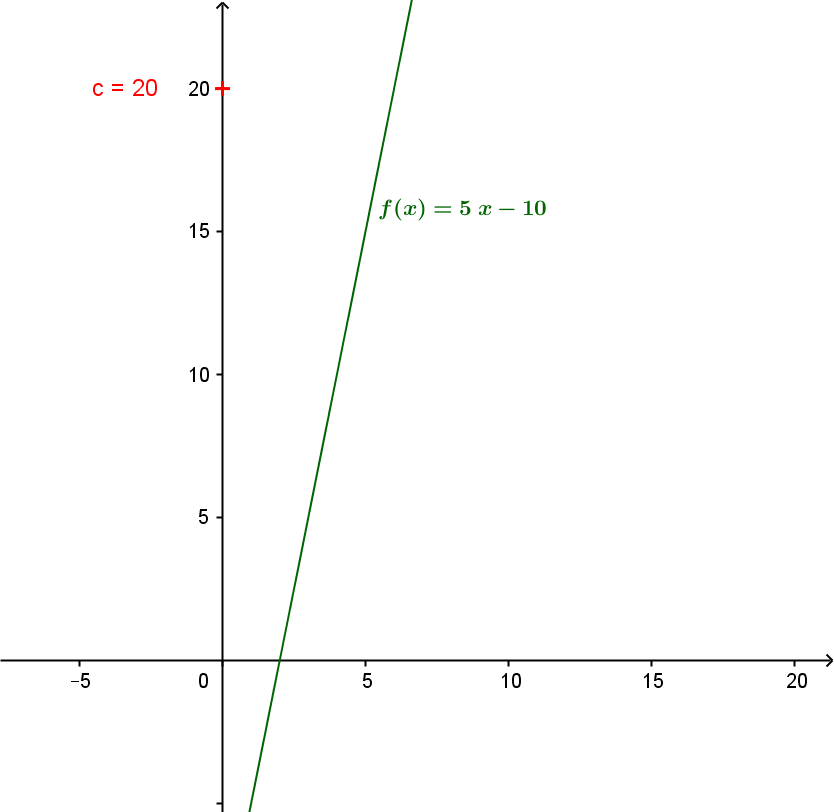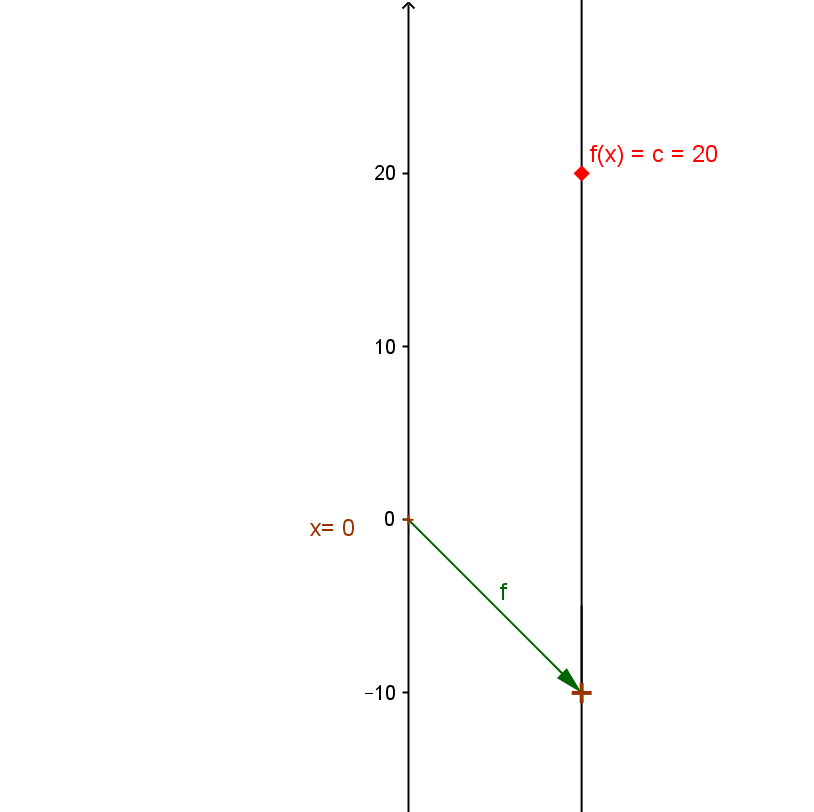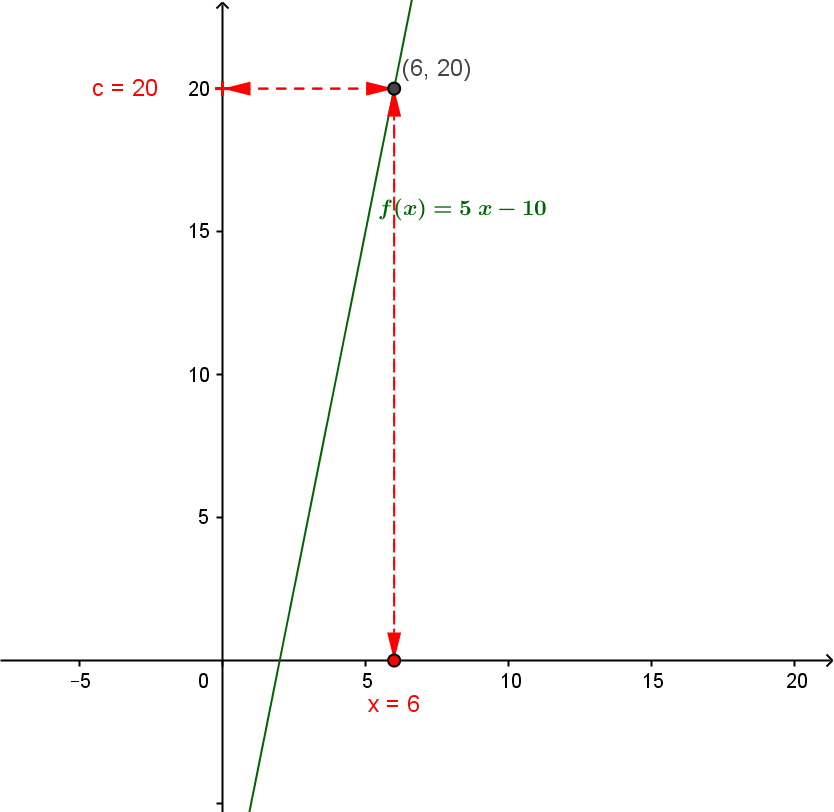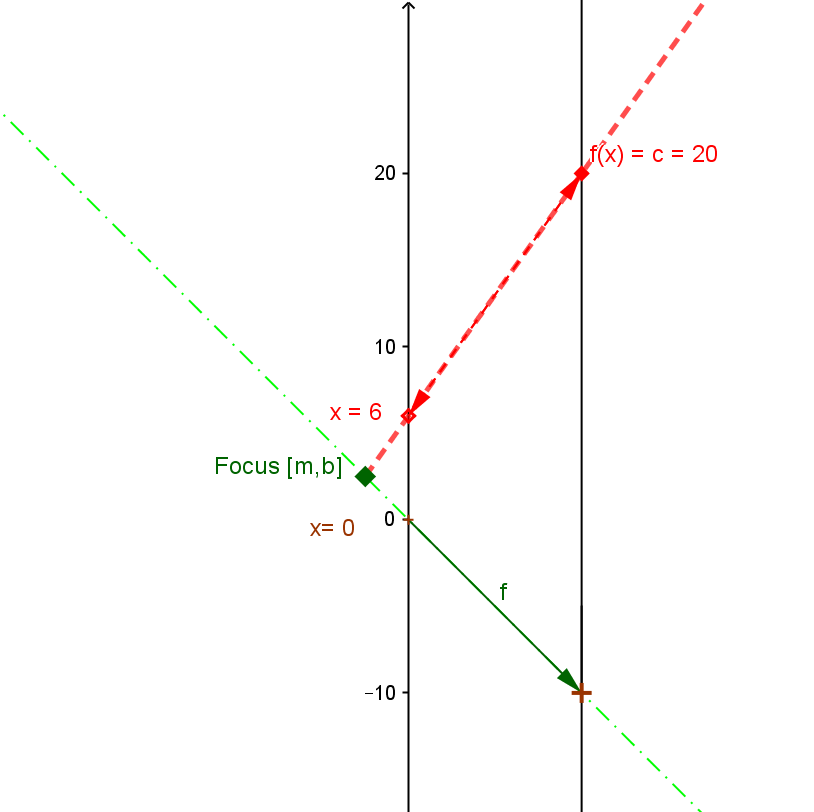Example  1.  Suppose $5x - 10 = 20$. Find $x$.
Solution: Since $A \ne 0$, the equation can be solved so that $x = \frac {C- B} A$  where $A=5, B = -10$, and $C = 20$. So the solution is $x = \frac {20 - (-10)} 5 = \frac {30} 5 = 6.$

Comment: We can consider the expression on the left hand side of the equation as a function of $x$ giving $f(x) = 5x - 10$ . Now the problem can be restated: to find a $x$ where $f(x) = 20$. This problem and its solution can be visualized both on the graph and the mapping diagram for the function $f$.

See also Example LEQ.3 for a visual approach to solving a linear equation that parallels the usual algebraic steps.

 For the graph of $f$: Find $y=20$ on the Y axis , then find the point on the graph of $f$ with second coordinate $20$, determine it's first coordinate, $6$, and that is the desired value for $x$. For the mapping diagram of $f$: Find $y=20$ on the target axis , then find $x$ on the source axis with the function arrow pointing to $20$.To do this, look for the point where the line $y=20$ intersects the line that is the graph of $f$ To do this,  find the focus point of $f$, $F= [5, -10]$ on the mapping diagram. [Use Geogebra?] Draw the line through F and the point $y=20$, to find the point of intersection of this line with the X axis, $x=6$, which is the desired value for $x$.See also Example LEQ.3 for a visual approach to solving a linear equation that parallels the usual algebraic steps.